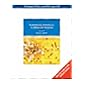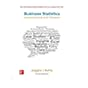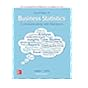Normal view MARC view ISBD view

# Business statistics : communicating with numbers / Sanjiv Jaggia, Alison Kelly.

Call number: 519.5 J24B Material type:BookPublisher: New York, N.Y. : McGraw-Hill, c2013. Edition: International ed.Description: xxxi, 714 p. : ill.ISBN: 9780071317610 (pbk.).
Contents:
Part 1. Introduction. 1, Statistic and data -- Part 2. Descriptive statistics. 2, Tabular and graphical methods. 3, Numerical descriptive measures -- Part 3. Probability and probability distributions. 4, Introduction to probability. 5, Discrete probability distributions. 6, Continuous probability distributions -- Part 4. Basic inference. 7, Sampling and sampling distributions. 8, Estimation. 9, Hypothesis testing. 10, Statistical inference concerning two populations. 11, Statistical inference concerning variance. 12, Chi-square tests -- Part 5. Advance inference. 13, Analysis of variance. 14, Regression analysis. 15, Inference with regression models. 16, Regression models for nonlinear relationships. 17, Regression models with dummy variables -- Part 6. Supplementary topics. 18, Time series and forecasting. 19, Returns, index numbers, and inflation. 20, Nonparametric tests -- Appendixes. A, Tables. B, Answers to even-numbered exercises.
Tags from this library: No tags from this library for this title. Log in to add tags.CL_General Book 519.5 J24B (Browse shelf) Available 1 1000172683No cover image available 519.5 H859F 1999 Fundamental statistics for the behavioral sciences 519.5 H859F 2004 Fundamental statistics for the behavioral sciences 519.5 H859F 2008 Fundamental statistics for the behavioral sciences 519.5 J24B Business statistics : 519.5 J24B 2019 Business statistics : 519.5 J24E 2020 Essentials of business statistics : 519.5 J58S Statistical survey techniques